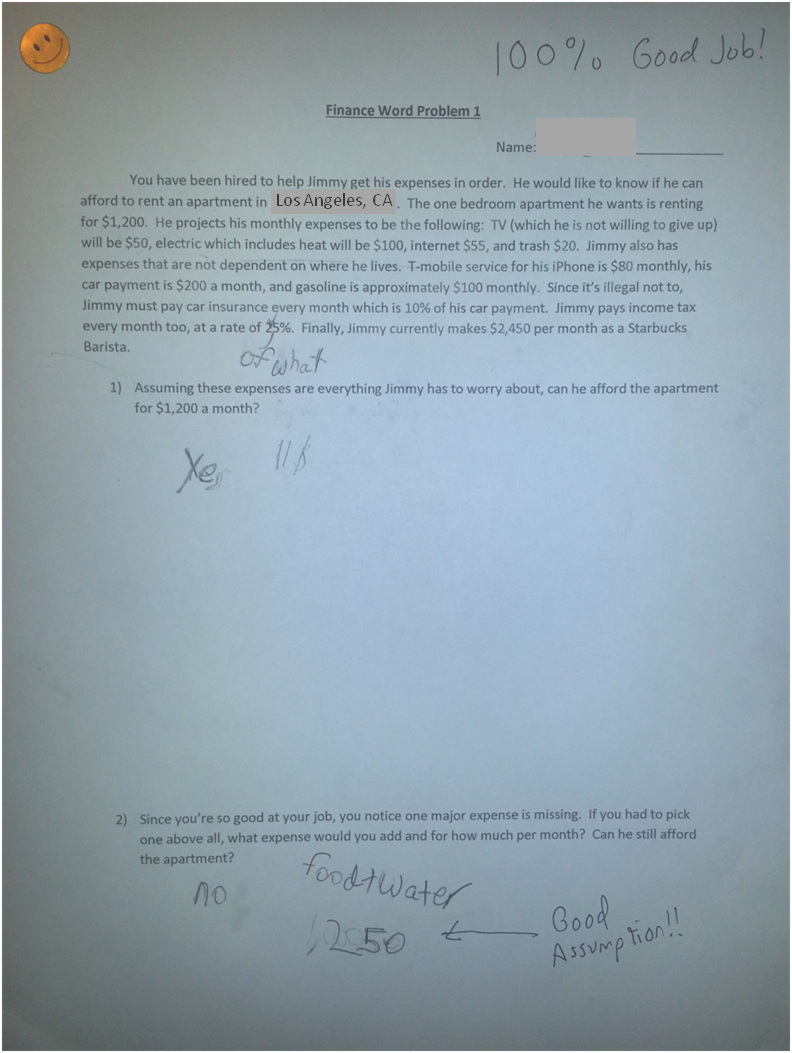# What Makes a Good Math Word Problem?

Our son was showing signs of interest in finance, business, and investing so we wanted to provide him with a good, practical, mathematical word problem.  We started a series of problems that focus on finance and the first one we gave him was a basic lesson in income and expenses.   This problem is a great exercise for a number of reasons.  First, it teaches fiscal responsibility and gives the student an idea of what certain essentials actually cost, something that is not highly focused on in most elementary education and perhaps should be.  Second, it causes the student to keep track of the information in the problem, perhaps by use of a table with two columns:  income and expenses (our son used a separate sheet of paper).   Third, it empowers the student to help the character described in the narrative (Jimmy in this case) and provides relevance as the student is applying skills relative to a job they have been hired for.  Fourth, it asks practical questions that force the student to think, generate original ideas, and compare or test those ideas.  The first question doesn’t simply ask a rote math question such as “What is the sum?” or “How much is left?”  It asks the student if Jimmy can afford something which forces a comparison of numbers that are found only after a number of proper calculations.  The second question is great because it forces the student to think critically, gives them freedom to decide, and causes a change in the outcome.  Additionally, there is no wrong answer.  In this case, our son advises that Jimmy forgot to include “food and water” in his calculations and assumes it will cost \$250 per month, which is reasonable.  This problem can extend to other mathematical applications as well—use your imagination!  From this exercise and more like it, a student can practice basic math, but also keep track of information, make needed comparisons, and provide empowering advice!